## Понедельник 17.10. Krzysztof Sornat: "Approximation and Parameterized Complexity of Minimax Approval Voting"

Понедельник, 17 октября, ауд. 106. Начало в 14:00.

Докладчик: Krzysztof Sornat (University of Wroclaw).

Тема: Approximation and Parameterized Complexity of Minimax Approval Voting.

### Abstract

We present three results on the complexity of Minimax Approval Voting that is a voting rule for choosing committee of fixed size k. Here, we see the votes and the choice as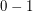strings of length(characteristic vectors of the subsets). The goal is to minimize the maximum Hamming distance to a vote. First, we study Minimax Approval Voting parameterized by the Hamming distance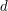from the solution to the votes. We show Minimax Approval Voting admits no algorithm running in time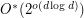, unless the Exponential Time Hypothesis (ETH) fails. This means that the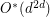algorithm of Misra et al. [AAMAS 2015] is essentially optimal. Motivated by this, we then show a parameterized approximation scheme, running in time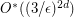, which is essentially tight assuming ETH. Finally, we get a new polynomial-time randomized approximation scheme for Minimax Approval Voting, which runs in time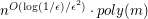, almost matching the running time of the fastest known PTAS for Closest String due to Ma and Sun [SIAM J. Comp. 2009].

Authors: Marek Cygan, Łukasz Kowalik, Arkadiusz Socała, Krzysztof Sornat
http://arxiv.org/abs/1607.07906